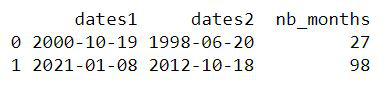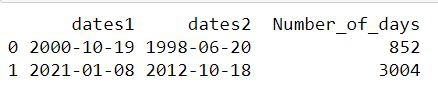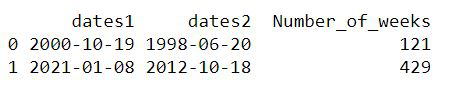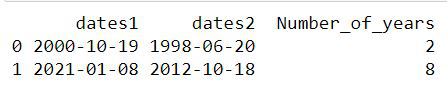GFG App
Open AppBrowser
Continue

# Pandas – Number of Months Between Two Dates

In this article, we are going to find the number of months between two dates in pandas using Python.

Example 1:

We will take a dataframe and have two columns for the dates between which we want to get the difference. Use df.dates1-df.dates2 to find the difference between the two dates and then convert the result in the form of months. Convert into ‘int’ datatype else result will be in form of float.

## Python3

 `# Importing required libraries` `import` `pandas as pd` `import` `numpy as np` `import` `datetime`   `# Making a dataframe which will have two` `# columns two store different dates` `df ``=` `pd.DataFrame({``'dates1'``: np.array(` `  ``[datetime.datetime(``2000``, ``10``, ``19``), datetime.datetime(``2021``, ``1``, ``8``)]),` `                   ``'dates2'``: np.array(` `                     ``[datetime.datetime(``1998``, ``6``, ``20``),` `                      ``datetime.datetime(``2012``, ``10``, ``18``)])})`   `# Used to convert the difference in terms of months` `df[``'nb_months'``] ``=` `((df.dates1 ``-` `df.dates2)``/``np.timedelta64(``1``, ``'M'``))` `df[``'nb_months'``] ``=` `df[``'nb_months'``].astype(``int``)` `print``(df)`

Output:Example 2:

We can also get the number of days between two dates by doing a slight modification in the code. It is shown as follows:

## Python3

 `# Importing required libraries` `import` `pandas as pd` `import` `numpy as np` `import` `datetime`   `# Making a dataframe which will have` `# two columns two store different dates` `df ``=` `pd.DataFrame({``'dates1'``: np.array(` `  ``[datetime.datetime(``2000``, ``10``, ``19``), datetime.datetime(``2021``, ``1``, ``8``)]),` `                   ``'dates2'``: np.array(` `                     ``[datetime.datetime(``1998``, ``6``, ``20``),` `                      ``datetime.datetime(``2012``, ``10``, ``18``)])})`   `# Used to convert the difference in terms of days` `df[``'Number_of_days'``] ``=` `((df.dates1 ``-` `df.dates2)``/``np.timedelta64(``1``, ``'D'``))` `df[``'Number_of_days'``] ``=` `df[``'Number_of_days'``].astype(``int``)` `print``(df)`

Output:Example 3:

In a similar way, we can get differences between the two dates in terms of weeks also.

## Python3

 `# Importing required libraries` `import` `pandas as pd` `import` `numpy as np` `import` `datetime`   `# Making a dataframe which will` `# have two columns two store different dates` `df``=` `pd.DataFrame({``'dates1'``: np.array(` `  ``[datetime.datetime(``2000``, ``10``, ``19``), datetime.datetime(``2021``, ``1``, ``8``)]), ` `                   ``'dates2'``: np.array(` `                     ``[datetime.datetime(``1998``, ``6``, ``20``),` `                      ``datetime.datetime(``2012``, ``10``, ``18``)] )})`   `# Used to convert the difference in terms of weeks` `df[``'Number_of_weeks'``] ``=` `((df.dates1 ``-` `df.dates2)``/``np.timedelta64(``1``, ``'W'``))` `df[``'Number_of_weeks'``] ``=` `df[``'Number_of_weeks'``].astype(``int``)` `print``(df)`

Output:Example 4:

In a similar way, we can get differences between the two dates in terms of years also.

## Python3

 `# Importing required libraries` `import` `pandas as pd` `import` `numpy as np` `import` `datetime`   `# Making a dataframe which will` `# have two columns two store different dates` `df ``=` `pd.DataFrame({``'dates1'``: np.array(` `  ``[datetime.datetime(``2000``, ``10``, ``19``), datetime.datetime(``2021``, ``1``, ``8``)]),` `                   ``'dates2'``: np.array(` `                     ``[datetime.datetime(``1998``, ``6``, ``20``), ` `                      ``datetime.datetime(``2012``, ``10``, ``18``)])})`   `# Used to convert the difference in terms of years` `df[``'Number_of_years'``] ``=` `((df.dates1 ``-` `df.dates2)``/``np.timedelta64(``1``, ``'Y'``))` `df[``'Number_of_years'``] ``=` `df[``'Number_of_years'``].astype(``int``)` `print``(df)`

Output:My Personal Notes arrow_drop_up